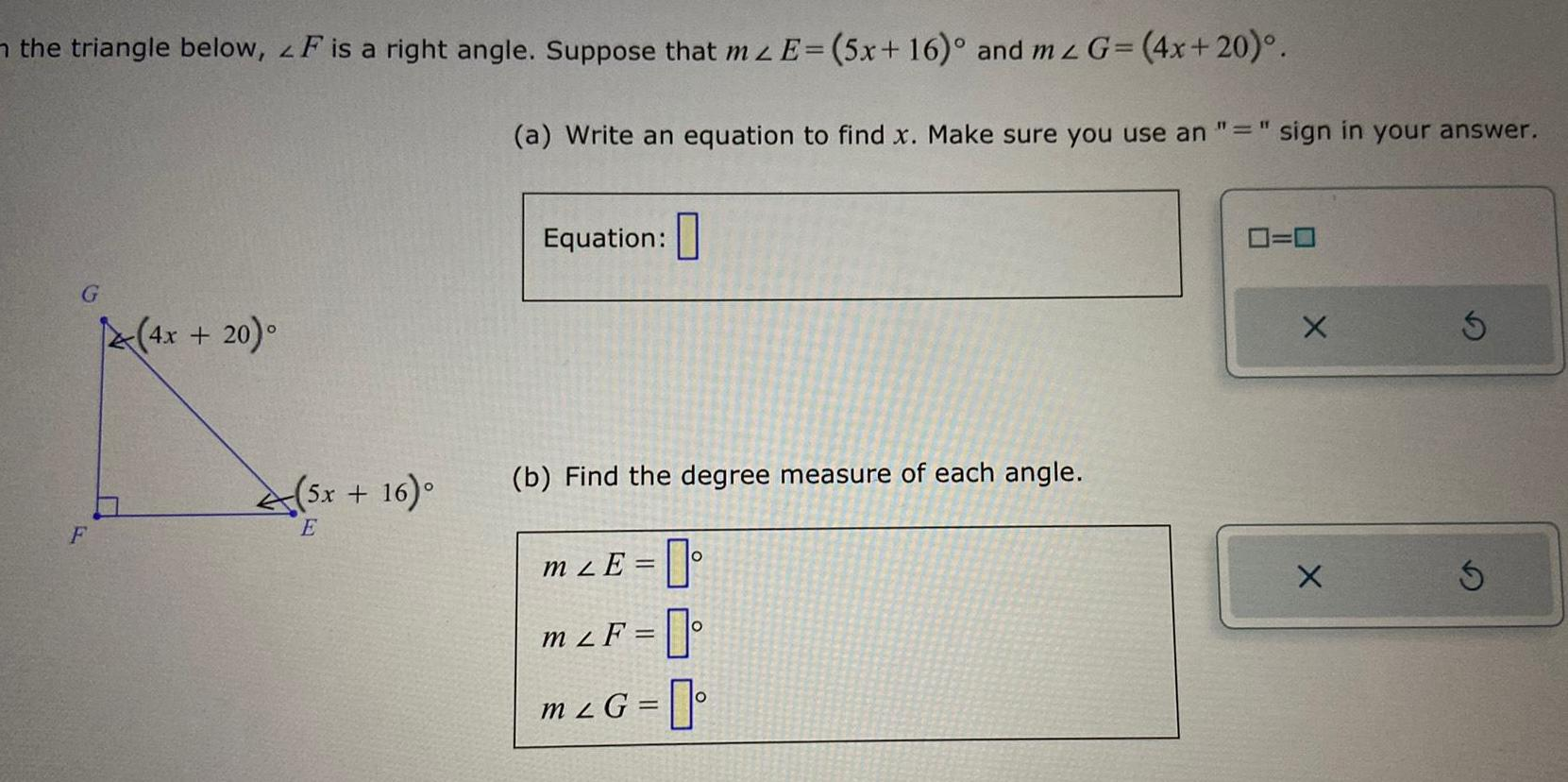Question:

# the triangle below LF is a right angle Suppose that mz E 5x

Last updated: 9/16/2023the triangle below LF is a right angle Suppose that mz E 5x 16 and mz G 4x 20 G F 4x 20 45x 16 E a Write an equation to find x Make sure you use an sign in your answer Equation b Find the degree measure of each angle mLE 0 mLF m G 0 0 X X S